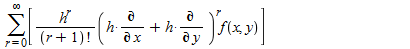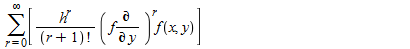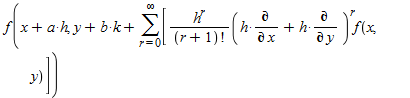## Problem with involution...

Hi everyone,

Consider this maple 18 doc: Euler18.mw

The code is regular code for Julia sets of the exponential.

To see how the Julia set behaves at infinity, I apply the transform mu(z)=1/z.

The plot3d command correctly plots the Julia set at an appropriate neighborhood of infinity, but:

1) Axes are not transformed

2) Saving as .eps produces an empty plot, sans the axes (plot is saved correctly, when not applying mu(z))

Is there any trick to force the axes to also show correctly with the transformed ranges?

Seems that this misalignment is bothering the .eps renderer, which probably plots the graph in twilight zone, given the false ranges of the untransformed axes.

Any ideas on how to force the saveas .eps to work in this case?

Many thanks,

Yiannis

## How to evaluate the double integral?...

I would like to plot the following singular double integral, but I cannot due to singularities...where x>0, t=0.2 and m=0.2.

I defined f(y) function as f:=y->exp(-(y-4.68)^2/0.4):

I attached my file:
1st_try.mw

Thank you !

## How to solve that ODE?...

i have this problem -> f'^2 -ff''=f'''-k1(2f'f'''-ff''''-f''^2)+Ha^2(E1-f') with boundary conditions f(0)=0, f'(0)=1, f'(∞)=0.

since it is a fourth order equation, but only three bcs, it does not produce unique solution. so the solution of the equation may be seek in form of f=f0(eta)+k1f1(eta).

thus the equation will become

f0'^2-f0f0''=f0'''+Ha^2(E1-f0')

and

f1'''-Ha^2f1'-2f0'f1'+f0f1''+f1f0''=2f0'f0'''-f0f0''''-f0''^2.

boundary conditions are

f0(0)=0,f0'(0)=1,f0'(∞)=0

f1(0)=0,f1'(0)=0,f1'(∞)=0.

i had been clueless in solving this problem. please somebody help me with this problem.

## How to solve that recursion?...

Given the sequence defined by the recursive relation a[n+1] = r*a[n](1-a[n])
You need to use the procedure iterate.
Throughout this problem you should choose initial values in the interval 0<a0<1.
(a) Let r=3/2. Calculate a moderate number of terms in the sequence (between 10 and 20). Does the sequence appear to be converging? If so to what value? Does the limit depend upon your choice of initial value? Plot the terms you have calculated
(b) Let r=2.8. Calculate a moderate number of terms in the sequence (between 10 and 20). Does the sequence appear to be converging? If so to what value? Does the limit
depend upon your choice of initial value? Plot the terms you have calculated How does this sequence differ from that in part (a).
(c) Let r=3.2. Calculate a moderate number of terms in the sequence (between 10 and 20). Show that the sequence does not appear to converging. Plot the terms you have calculated and describe how the sequence behaves in this case.
(d) Consider intermediate values between 2.8 and 3.2 to determine more precisely where the transition in behaviour takes place. Provide a few plots (no more than 4) showing the values you have investigated.
(e) Consider the values of r in the range 3.43<r<3.46. Determine as accurately as you can the value of r for which the period of oscillation doubles.
(f) As r increase further period doubling occurs. Try to find the when the sequence appears to oscillate between 8 values.
(g) Let r =3.65 and calculate a considerable number of terms (at least a few hundred) and plot your values.
(h) For r=3.65 choose a0=0.3 and then a0=0.301. Find and plot some terms in the sequence for each initial value. Determine how long the terms in the two sequences remain close together and when they begin to depart significantly from each other.

## Putting error bars on curve fitting graph...

I have used data and the curve fitting procedure to find a curve and I now have to put error bars on the graph to complete my task? I know that there is an errorplot function but I cant seem to find a way to do it with the curvefitting thing?

I have attached the whole worksheet, I hope someone can help! Thanks Detectors_Tutorial.mwDetectors_Tutorial.mw

Even if there was some method of exporting the graph to another medium that would also be very helpful

## Fit routine to find parameters...

Hello,

I am pretty new to Maple and Im trying to find the parameters of an equation using some kind of fit routine but I can only find such a routine to fit an expression to data and not vice versa.

my equation is as follows

epsilon(E):= a0 + a1*ln(E) + a2*ln(E)^2 + a3*ln(E)^3

I do have data for E which I imagine I need.

E:= {121, 244, 344, 411, 444, 778, 867, 964, 1085, 1112, 1212, 1299, 1408} all in keV :)

Any suggestions/help would be much appreciated, although I am new to the program so go easy on me :)

Cheers

## How to select eigenvalue?...

Hi Mr Preben Alsholm .

for determine unknown parameter oemega(or eigenvalue) according to line ''newsys2 := subs(omega^2 = omega2*10^5,newsys)'' and also line ''approxsoln = [omega2 = 1, f(x) = x^2*(1-x)^2]''

power (5) in term'' 10^5''  in  (omega2*10^5,newsys) and initial guess (1)  (omega2 = 1) are very effective on final result eigenvalues .sometimes gain different eigenvalues ,which it is not impossible recognize that

which one is correct and convege.however i need Positive

eigenvalue that is minimum between them,If and only if , it converge after
some iteration in two section of maple file which is attached as
Eigenvalue.mw

According to my code converge occuerd until  eigenvalue gained in first section (before line `11` := ((1+6*alpha2)*(1/12))*(int(fy11^2, x = 0 .. 1))...........) are equal with those obtain in second section .(please see below file for example for_example.mw)

it is necessary mention that between section 1 and 2 is relations.for example amont of first obtained eigenvalue which obtain from first section must be repleaced in line ''approxsoln = [omega2 = 0.661514014001420, h(theta) = theta^2*(1-theta)^2]...........''in second section,

and this procedure should be continued between section 1 and 2 until convergence with desired accuracy occurred.Another relate is that,the first ODE system  can be solved using the first set of boundary data to obtain first estimate for  second section (fy11). At the next step by repeating in the same manner,

this time by obtained function (g) at the end of maple code (Solution of the second ODE system  with second set of boundary conditions leads to the first estimate of function g),that at this stage, the first iteration is completed.

Now by replacing omega2 which is determined in this section in to the first section ,fy11 is updated and gained Further.

Next, the updated function , by continuing the iterative procedure.

in matlab bvp4c rule is used for this purpose.is this impossible in maple software ?if not please help me for solve and gain correct omega..

thanks alot

## Calculus assignment...

I am doing a Calculus assignment and I can't find the commands for certain things.

1.Given the function f(x) = ((x+1)^2) / (1+x^2)

i) The domain of continuity of f(x)

ii) The intervals of increase and decrease of f(x) by using test points.

2. Use the IVT to prove existence of a root to the equation x^3 +10x^2 -100x +50=0 in the interval [-20,10]. Use again the IVT to show that there is a 1st root in [-17,-15], a 2nd toot in [0,1] and a 3rd root in [ 5,6]. Find or approximate those roots with Maple. (the bolded is what I need help).

## Font size for printing worksheets from Maple 18...

Hi,

I am working on a Windows machine. In older Maple versions, decreasing the font size for the screen display (via zoom factor) also decreased the font size for the printout. But now the printout comes with a pretty large font size and does not change when using the zoom factor. It practically makes no sense anymore to print. How can I decrease the fonts for the printout to my liking?

Thanks.

heide

## Free boundary problem...

Hello altogether,

I want to plot the numerical result of an ODE, which seems to be pretty simple at first sight, but the difficulty is that the boundaries are depending on the solution.

The following pseudo-code describes what I want to have, but it doesn't work. This code fills the RAM pretty fast and you will have to kill the process.

Free-boundary_prob.mw

Is it possible to calculate a solution to this problem numerically (or even analytically) and if yes, how?

Since I am new here, I am sorry for any bad-to-read maple code or any noob errors I have made. I would be very thankful, for any response and help.

Greetings

butterflyfart

## Problem with dsolve...

thanks alotequation.mw

## How do I improve the performance of Maple 18 for s...

I am trying to use Maple 18 to do some computations with matrices over a ring of polynomials in one variable over the integers \$\mathbb{Z}[x]\$, or the corresponding field of fractions \$\mathbb{Q}(x)\$.

The matrices in question are of dimension approximately 5000 and are sparse. The algorithm requires at least as many matrix multiplications as the dimension of the space.

Doing some small examples, of dimension 674, with a laptop (i7-3520 M CPU @2.9GHz with 8GB of Ram) gave the following disappointing result:

time(LinearAlgebra[MatrixMatrixMultiply](A,A);

34.694

When a colleague with access to a Mathematica license performed an identical calculation using sparse matrices in Mathematica, we found that Mathematica performed the calcuation in fractions of a second.

In small dimensional examples, constructing the matrices over the field of fractions as sparse in Maple 18 resulted in a four fold decrease in the already disappointing performance of the LinearAlgebra package in Maple 18.

Is there any way to improve the computational performance of Maple 18 for symbolic linear algebra? Alternatively, is the performance of Maple 2015 for symbolic linear algebra noticably better than Maple 18?

Dave

## eBookTools ERROR...

Hi everyone,

I would like to create an eBook with the pakage "eBookTools", but I have got several errors if in my mw document there is a table. The same error came out if I try to compile a book with the sample documents ("GettingStartedWithMaple.mw"). Anyone knows a solution?

Giorgio

## How to expand these expressions?...andand